# Coordination Compounds - JEE Advanced Previous Year Questions with Solutions

JEE Advanced Previous Year Questions of Chemistry with Solutions are available at eSaral. Practicing JEE Advanced Previous Year Papers Questions of Chemistry will help the JEE aspirants in realizing the question pattern as well as help in analyzing weak & strong areas. Simulator Previous Years JEE Advance Questions
Q. The spin only magnetic moment value (in Bohr magneton units) of \mathrm{Cr}(\mathrm{CO})_{6} is (A) 0 (B) 2.84 (C) 4.90 (D) 5.92 [JEE 2009]
Ans. (A)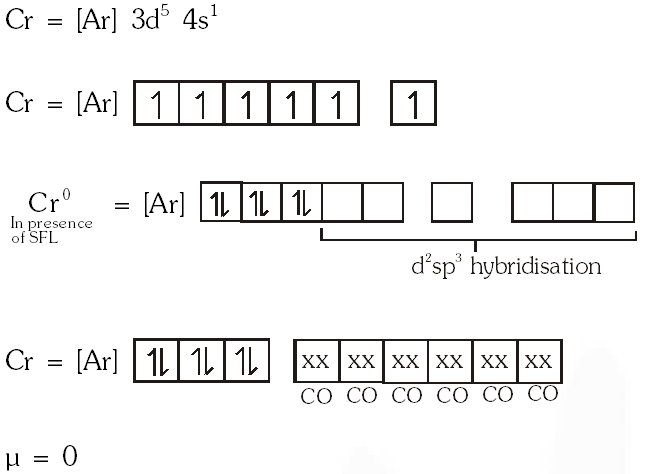Q. The compound(s) that exhibit(s) geometrical isomerism is (are) : (A) $\left[\mathrm{Pt}(\mathrm{en}) \mathrm{Cl}_{2}\right]$ (B) $\left[\mathrm{Pt}(\mathrm{en})_{2}\right] \mathrm{Cl}_{2}$ (C) $\left[\mathrm{Pt}(\mathrm{en})_{2} \mathrm{Cl}_{2}\right] \mathrm{Cl}_{2}$ (D) $\left[\mathrm{Pt}\left(\mathrm{NH}_{3}\right)_{2} \mathrm{Cl}_{2}\right]$ [JEE 2009]
Ans. (C,D)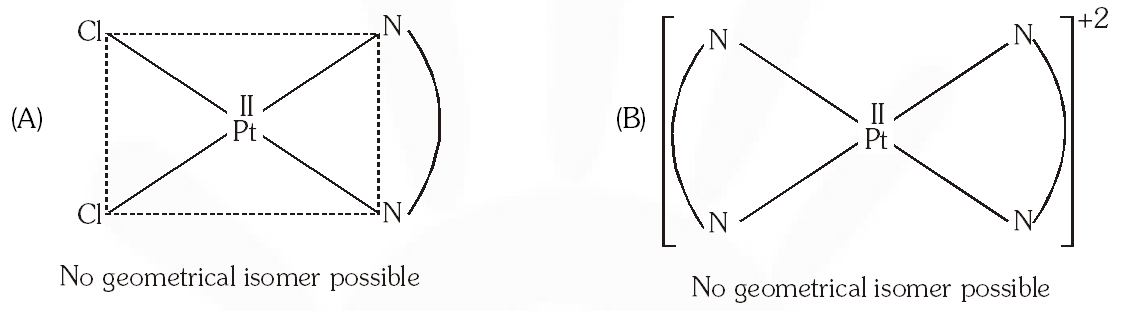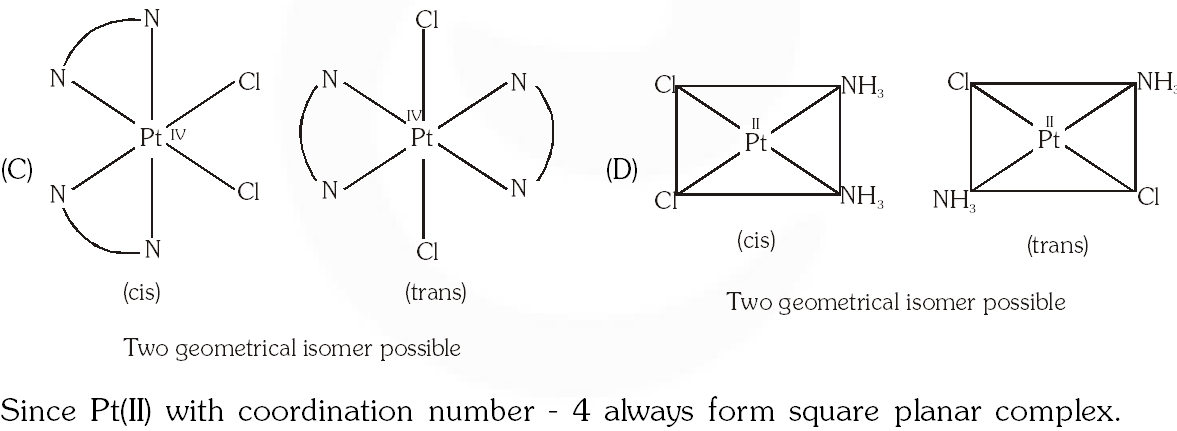Q. The number of water molecule(s) directly bonded to the metal centre in $\mathrm{CuSO}_{4}$. $5 \mathrm{H}_{2} \mathrm{O}$ is. [JEE 2009]
Ans. 4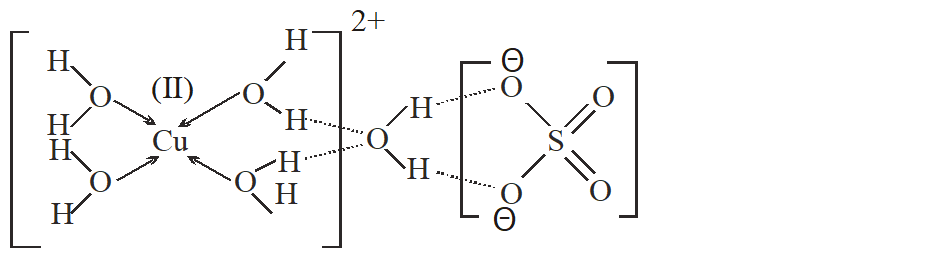Q. The ionization isomer of $\left[\mathrm{Cr}\left(\mathrm{H}_{2} \mathrm{O}\right)_{4} \mathrm{Cl}\left(\mathrm{NO}_{2}\right)\right] \mathrm{Cl}$ is – (A) $\left[\mathrm{Cr}\left(\mathrm{H}_{2} \mathrm{O}\right)_{4}\left(\mathrm{O}_{2} \mathrm{N}\right)\right] \mathrm{Cl}_{2}$ (B) $\left[\mathrm{Cr}\left(\mathrm{H}_{2} \mathrm{O}\right)_{4} \mathrm{Cl}_{2}\right]\left(\mathrm{NO}_{2}\right)$ (C) $\left[\mathrm{Cr}\left(\mathrm{H}_{2} \mathrm{O}\right)_{4} \mathrm{Cl}(\mathrm{ONO})\right] \mathrm{Cl}$ (D) $\left[\mathrm{Cr}\left(\mathrm{H}_{2} \mathrm{O}\right)_{4} \mathrm{Cl}_{2}\left(\mathrm{NO}_{2}\right)\right] \cdot \mathrm{H}_{2} \mathrm{O}$
Ans. (B) Ionisation isomers differ in ions in solution thus, ionisation isomer of $\left[\mathrm{Cr}\left(\mathrm{H}_{2} \mathrm{O}\right)_{4} \mathrm{Cl}\left(\mathrm{NO}_{2}\right)\right] \mathrm{Cl}$ is $\left[\mathrm{Cr}\left(\mathrm{H}_{2} \mathrm{O}\right)_{4} \mathrm{Cl}_{2}\right]\left(\mathrm{NO}_{2}\right)$. Because $\left[\mathrm{Cr}\left(\mathrm{H}_{2} \mathrm{O}\right)_{4} \mathrm{Cl}\left(\mathrm{NO}_{2}\right)\right] \mathrm{Cl} \longrightarrow\left[\mathrm{Cr}\left(\mathrm{H}_{2} \mathrm{O}\right)_{4} \operatorname{Cl}\left(\mathrm{NO}_{2}\right)\right]^{+}+\mathrm{Cl}^{-}$ (Given compound) $\left[\mathrm{Cr}\left(\mathrm{H}_{2} \mathrm{O}\right)_{4} \mathrm{Cl}_{2}\right]\left(\mathrm{NO}_{2}\right) \longrightarrow\left[\mathrm{Cr}\left(\mathrm{H}_{2} \mathrm{O}\right)_{4} \mathrm{Cl}_{2}\right]^{+}+\mathrm{NO}_{2}^{-}$ Ionisation isomer of given compound.
Q. Total number of geometrical isomers for the complex $\left[\mathrm{RhCl}(\mathrm{CO})\left(\mathrm{PPh}_{3}\right)\left(\mathrm{NH}_{3}\right)\right]$ is. [JEE 2010]
Ans. 3 $\left[\mathrm{RhCl}(\mathrm{Co})\left(\mathrm{PPh}_{3}\right)\left(\mathrm{NH}_{3}\right)\right]$ $\mathrm{dsp}^{2},$ square planar, total 3 geometrical isomer.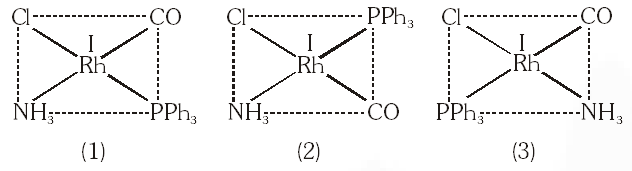Q. The correct structure of ethylenediaminetetraacetic acid (EDTA) is –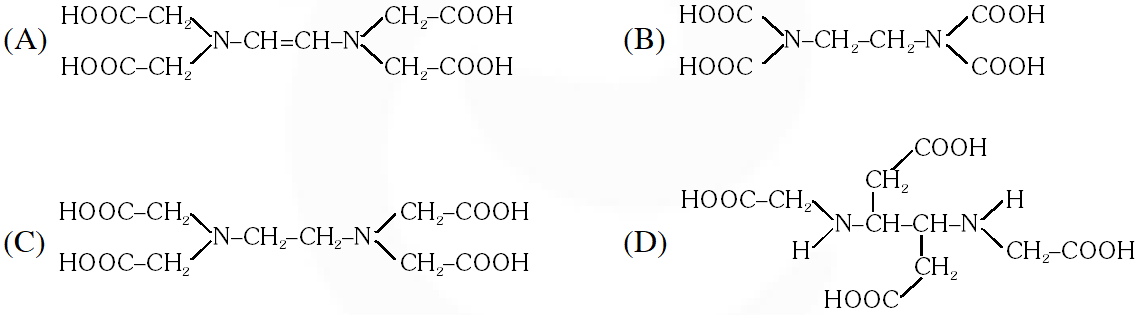[JEE 2010]
Ans. (C) The correct structure of ethylenediaminetetra acetic acid (EDTA) is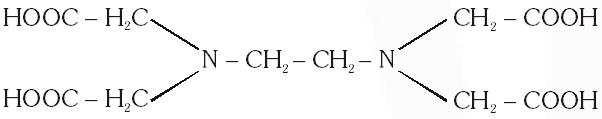Q. Geometrical shapes of the complexes formed by the reaction of $\mathrm{Ni}^{2+}$ with $\mathrm{Cl}^{-}, \mathrm{CN}$ and $\mathrm{H}_{2} \mathrm{O}$ respectively, are – (A) octahedral, tetrahedral and square planar (B) tetrahedral, square planar and octahedral (C) square planar, tetrahedral and octahedral (D) octahedral, square planar and octahedral [JEE 2011]
Ans. (B)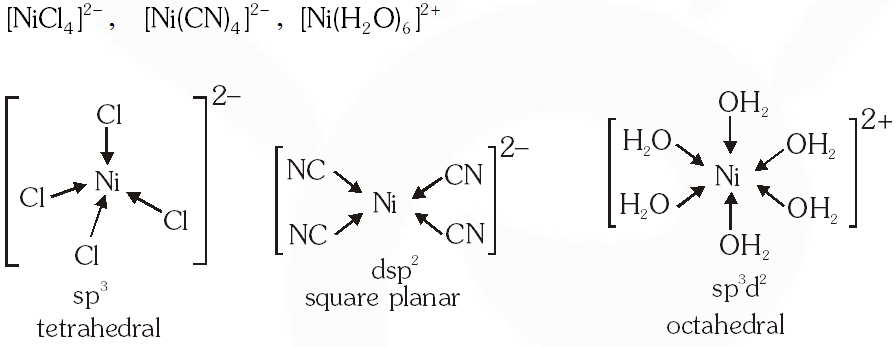Q. Among the following complexes (K–P) $\mathrm{K}_{3}\left[\mathrm{Fe}(\mathrm{CN})_{6}\right](\mathbf{K}),\left[\mathrm{Co}\left(\mathrm{NH}_{3}\right)_{6}\right] \mathrm{Cl}_{3}(\mathrm{L}), \mathrm{Na}_{3}\left[\mathrm{Co}\left(\text { oxalate) }_{3}\right](\mathrm{M}),\left[\mathrm{Ni}\left(\mathrm{H}_{2}\mathrm{O}\right)_{6}\right] \mathrm{Cl}_{2}(\mathrm{N})\right.$$\mathrm{K}_{2}\left[\mathrm{Pt}(\mathrm{CN}) {4}\right](\mathbf{O})$ and $\left[\mathrm{Zn}\left(\mathrm{H}_{2} \mathrm{O}\right)_{6}\right]\left(\mathrm{NO}_{3}\right)_{2}(\mathbf{P})$ The diamagnetic complex are – (A) K, L, M, N               (B) K, M, O, P               (C) L, M, O, P                (D) L, M, N, O [JEE 2011]
Ans. (C)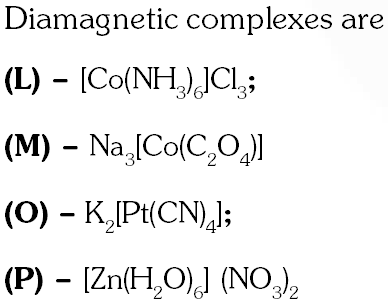Q. The volume (in mL) of 0.1M AgNO3 required for complete precipitation of chloride ions present in 30 mL of 0.01M solution of $\left[\mathrm{Cr}\left(\mathrm{H}_{2} \mathrm{O}\right)_{6} \mathrm{Cl}\right] \mathrm{Cl}_{2}$, as silver chloride is close to. [JEE 2011]
Ans. 6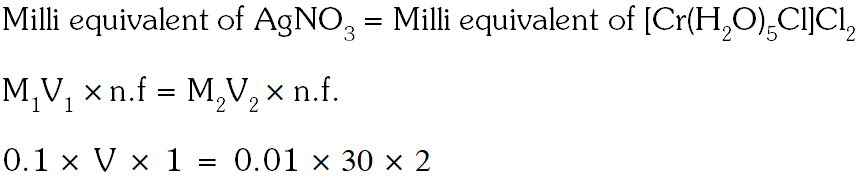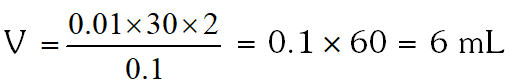Q. As per IUPAC nomenclature, the name of the complex $\left[\mathrm{Co}\left(\mathrm{H}_{2} \mathrm{O}\right)_{4}\left(\mathrm{NH}_{3}\right)_{2}\right] \mathrm{Cl}_{3}$ is : (A) Tetraaquadiaminecobalt(III) chloride (B) Tetraaquadiamminecobalt(III) chloride (C) Diaminetetraaquacobalt(III) chloride (D) Diamminetetraaquacobalt(III) chloride [JEE 2012]
Ans. (D) $\left[\mathrm{C}_{\alpha}\left(\mathrm{H}_{2} \mathrm{O}\right)_{4}\left(\mathrm{NH}_{3}\right)_{2} \mathrm{Cl}_{3}\right.$ Diamminetetraaquacobalt(III) chloride $\frac{V I B G Y O R}{\lambda-v^{-} E^{-}}$
Q. The colour of light absorbed by an aqueous solution of $\mathrm{CuSO}_{4}$ is – (A) orange-red (B) blue-green (C) yellow (D) violet [JEE 2012]
Ans. (A)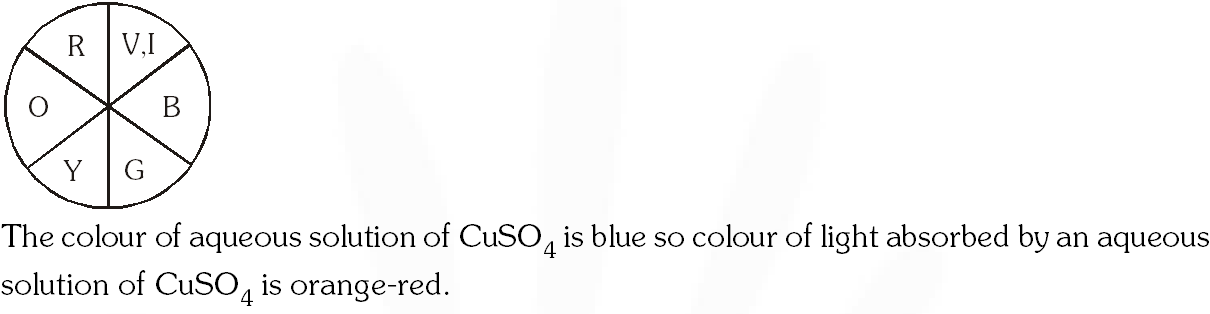Q. $\mathrm{NiCl}_{2}\left\{\mathrm{P}\left(\mathrm{C}_{2} \mathrm{H}_{5}\right)_{2}\left(\mathrm{C}_{6} \mathrm{H}_{5}\right)\right\}_{2}$ exhibits temperature dependent magnetic behavior (paramagnetic/diamagnetic). The coordination geometries of Ni2+ in the paramagnetic and diamagnetic states are respectively : (A) tetrahedral and tetrahedral (B) square planar and square planar (C) tetrahedral and square planar (D) square planar and tetrahedral [JEE 2012]
Ans. (C)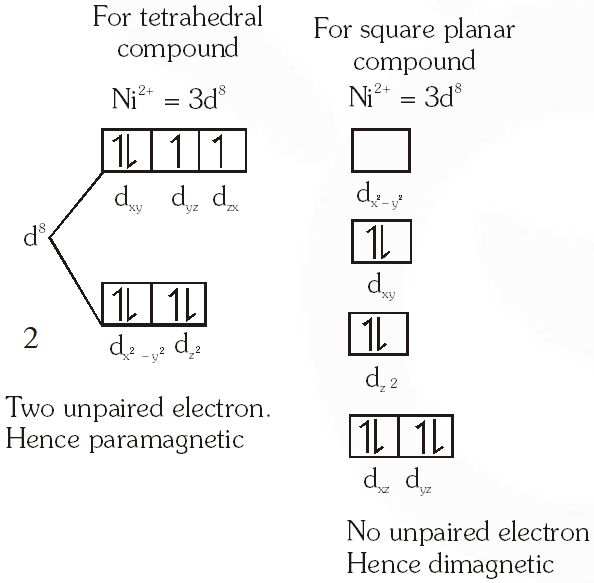Q. Consider the following complex ions P, Q and R , $\mathbf{P}=\left[\mathrm{FeF}_{6}\right]^{3-}, \mathbf{Q}=\left[\mathrm{V}\left(\mathrm{H}_{2} \mathrm{O}\right)_{6}\right]^{2+}$ and $\mathbf{R}=\left[\mathrm{Fe}\left(\mathrm{H}_{2} \mathrm{O}\right)_{6}\right]^{2+}$ The correct order of the complex ions, according to their spin-only magnetic moment values (in B.M.) is – (A) R < Q < P (B ) Q < R < P (C) R < P < Q (D) Q < P < R [JEE 2013]
Ans. (B)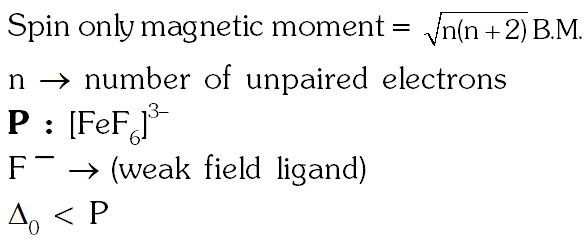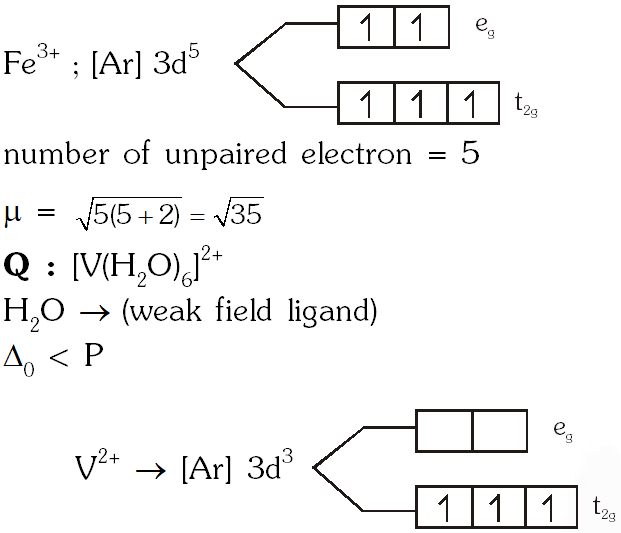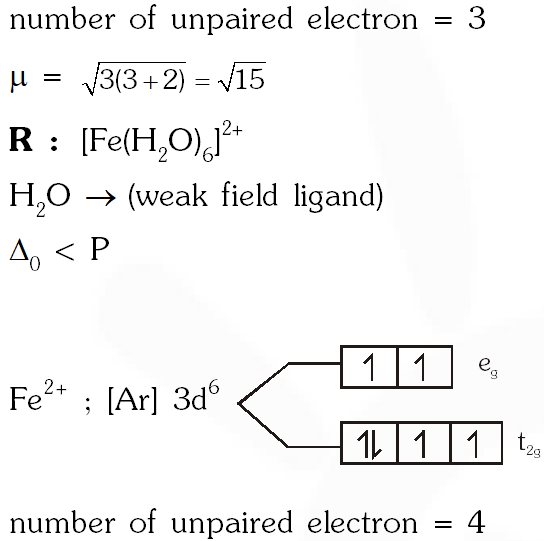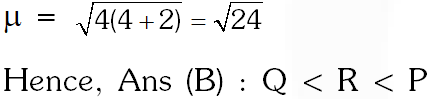Q. EDTA $^{4}$ is ethylenediaminetetraacetate ion. The total number of $\mathrm{N}-\mathrm{Co}-\mathrm{O}$ bond angles in $[\mathrm{Co}(\mathrm{EDTA})]^{-1}$ complex ion is - [JEE 2013]
Ans. 8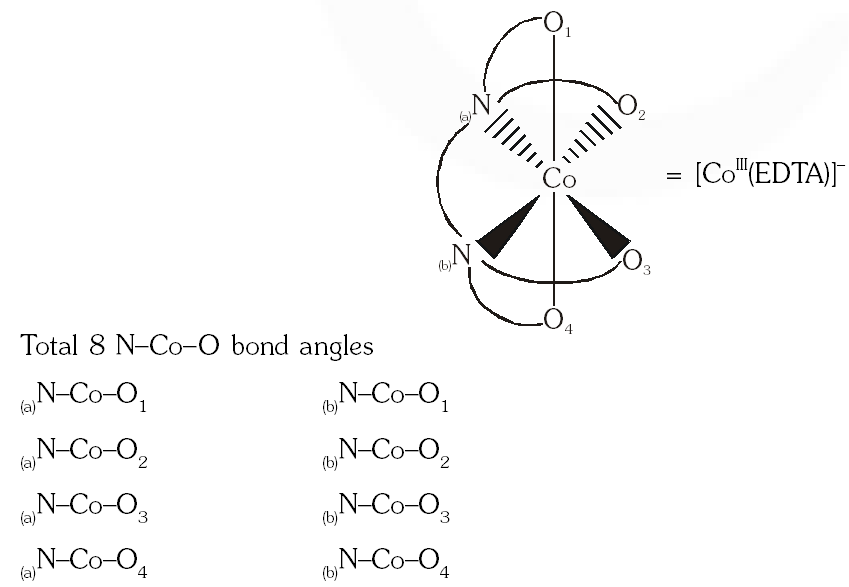Q. The pair(s) of coordination complex/ion exhibiting the same kind of isomerism is(are) – (A) $\left[\mathrm{Cr}\left(\mathrm{NH}_{3}\right)_{5} \mathrm{Cl}\right] \mathrm{Cl}_{2}$ and $\left[\mathrm{Cr}\left(\mathrm{NH}_{3}\right)_{4} \mathrm{Cl}_{2}\right] \mathrm{Cl}$ (B) $\left[\mathrm{Co}\left(\mathrm{NH}_{3}\right)_{4} \mathrm{Cl}_{2}\right]^{+}$ and $\left[\mathrm{Pt}\left(\mathrm{NH}_{3}\right)_{2}\left(\mathrm{H}_{2} \mathrm{O}\right) \mathrm{Cl}\right]^{+}$ (C) $\left[\mathrm{CoBr}_{2} \mathrm{Cl}_{2}\right]^{2-}$ and $\left[\mathrm{PtBr}_{2} \mathrm{Cl}_{2}\right]^{2-}$ (D)$\left[\mathrm{Pt}\left(\mathrm{NH}_{3}\right)_{3}\left(\mathrm{NO}_{3}\right)\right] \mathrm{Cl}$ and $\left[\mathrm{Pt}\left(\mathrm{NH}_{3}\right)_{3} \mathrm{Cl}\right] \mathrm{Br}$ [JEE 2013]
Ans. (B,D)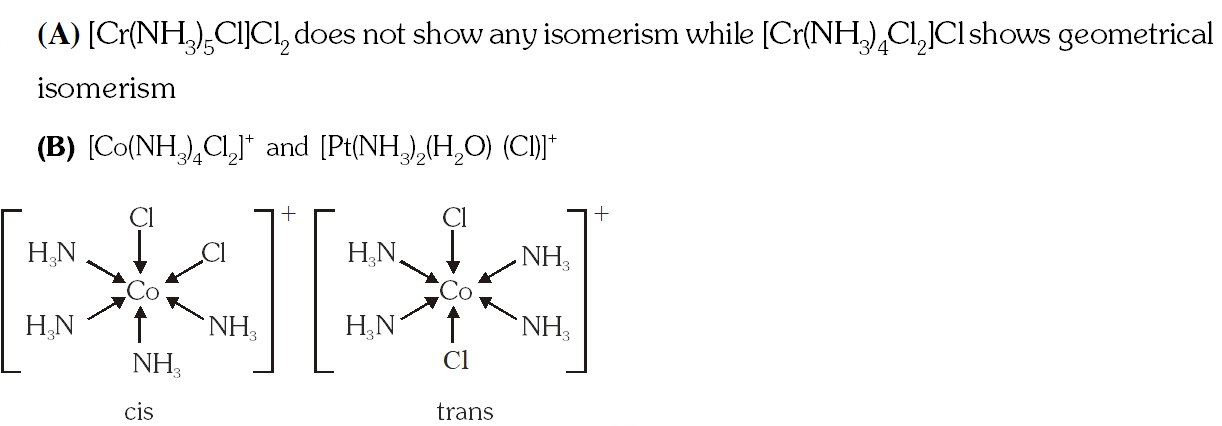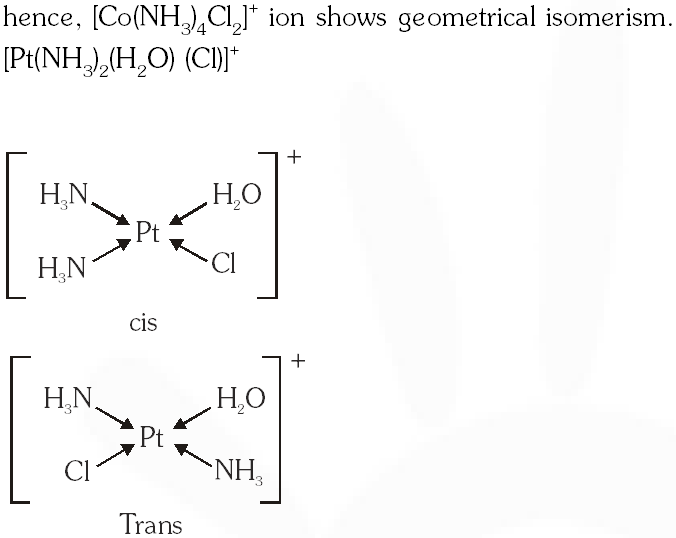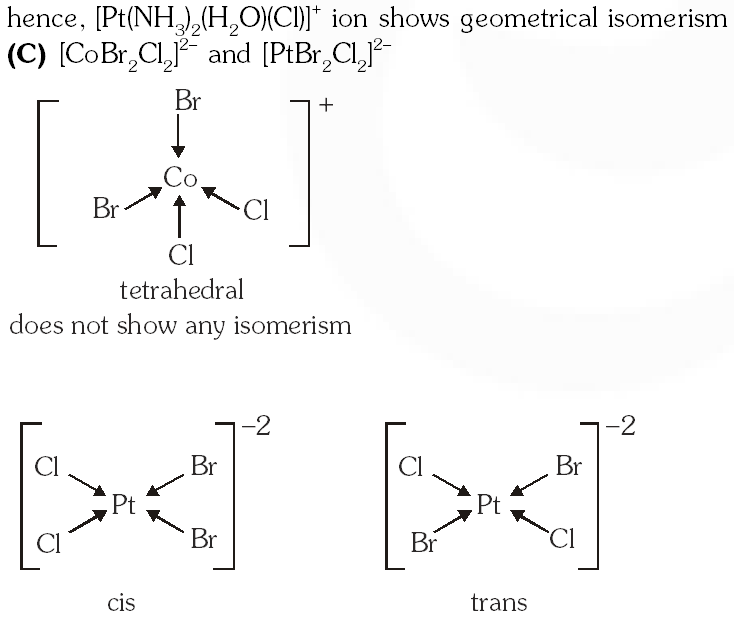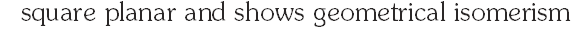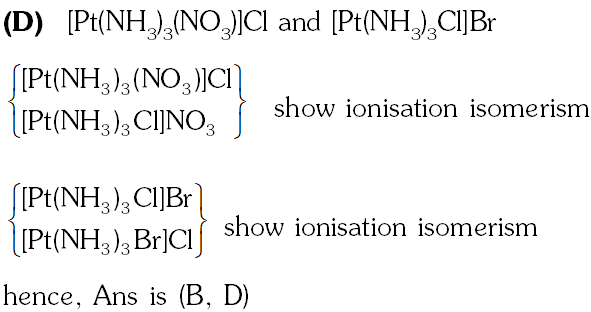Q. Match each coordination compound in List-I with an appropriate pair of characteristics from List-II and select the correct answer using the code given below the lists.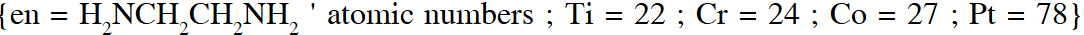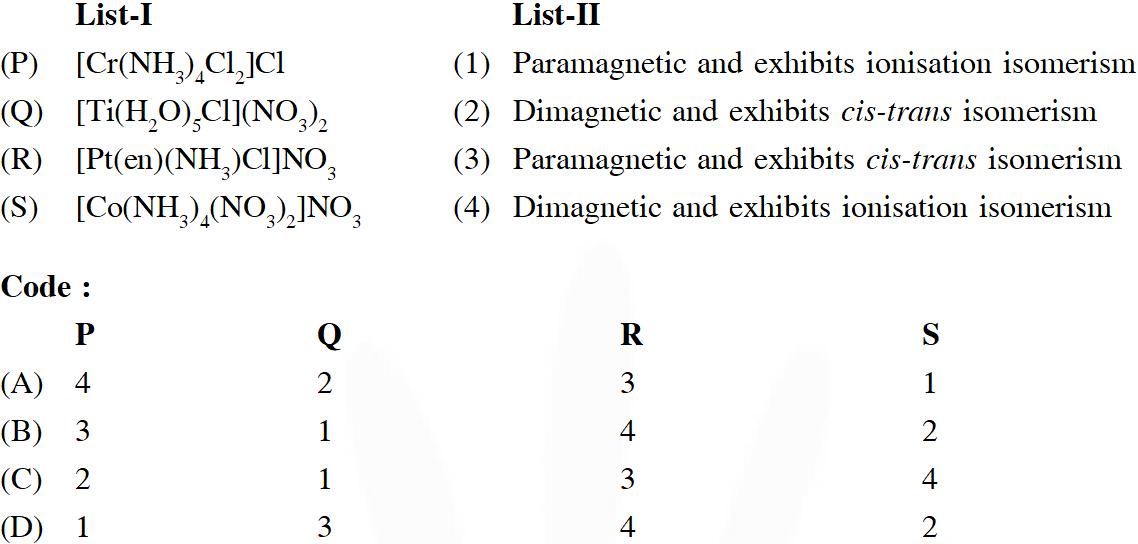[JEE Adv. 2014]
Ans. (B) (P) $\left[\mathrm{Cr}^{\mathrm{III}}\left(\mathrm{NH}_{3}\right)_{4} \mathrm{Cl}_{2}\right] \mathrm{Cl}:$ (1) Complex given in (P) is Paramagnetic & show two geometrical (3 unpaired electrons) isomerism (cis and trans) (does not show ionization isomer) (Q) $\left[\mathrm{Ti}^{\mathrm{III}}\left(\mathrm{H}_{2} \mathrm{O}\right)_{5} \mathrm{Cl}\right]\left(\mathrm{NO}_{3}\right)_{2}$ (2) Complex given in (Q) is paramagnetic show ionization(1 unpaired electrons) isomerism (R) $\left[\mathrm{Pt}^{\mathrm{Il}}(\mathrm{en})\left(\mathrm{NH}_{3}\right) \mathrm{Cl}\right] \mathrm{NO}_{3}$ (3) Complex given in (R) is diamagnetic and show ionization(1 unpaired electrons) isomerism (S)$\left[\mathrm{Co}^{\mathrm{III}}\left(\mathrm{NH}_{3}\right)_{4}\left(\mathrm{NO}_{3}\right)_{2}\right] \mathrm{NO}_{3}$ (4) Complex given in (S) is diamagnetic does not show ionization (0 unpaired electrons) isomerism show geometrical isomerism
Q.A list of species having the formula $\mathrm{XZ}_{4}$ is given below : $\mathrm{XeF}_{4}, \mathrm{SF}_{4}, \mathrm{SF}_{4}, \mathrm{BF}_{4}^{-}, \mathrm{BrF}_{4}^{-},\left[\mathrm{Cu}\left(\mathrm{NH}_{3}\right)_{4}\right]^{2+},\left[\mathrm{FeCl}_{4}\right]^{2-},\left[\mathrm{CoCl}_{4}\right]^{2-}$ and $\left[\mathrm{PtCl}_{4}\right]^{2-}$. Defining shape on the basis of the location of X and Z atoms, the total number of species having a square planar shape is [JEE Adv. 2014]
Ans.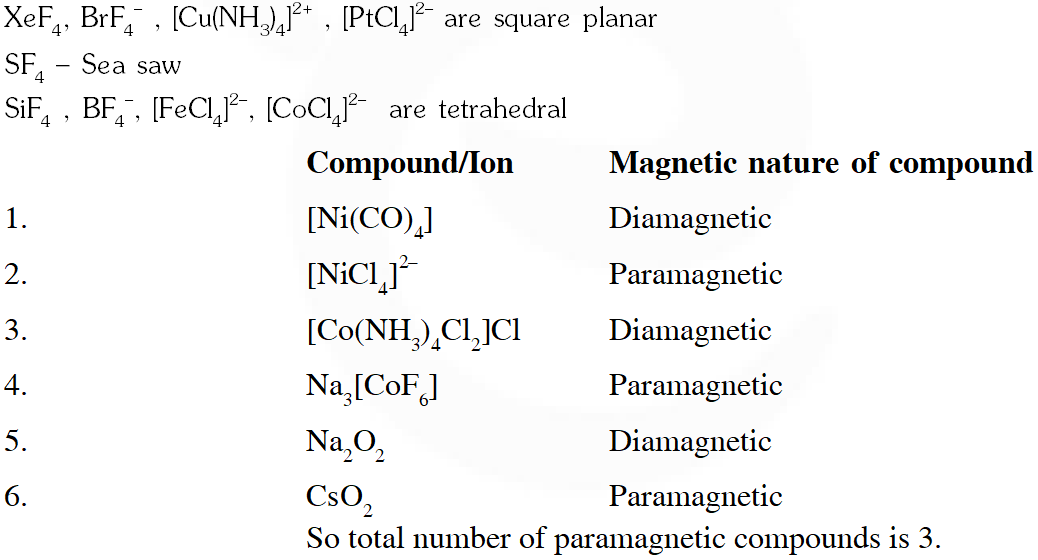Q. The geometries of the ammonia complexes of $\mathrm{Ni}^{2+}, \mathrm{Pt}^{2+}$ and $\mathrm{Zn}^{2+}$ , respectively , are : (A) octahedral, square planar and tetrahederal (B) square planar, octahederal and tetrahederal (C) tetrahederal, square planar and octahederal (D) octahederal , tetrahederal and square planar [JEE - Adv. 2016]
Ans. (A)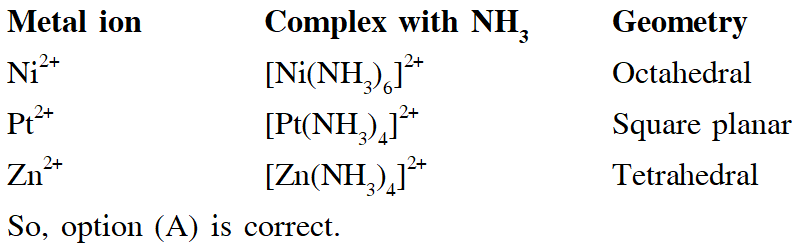Q. Among $\left[\mathrm{Ni}(\mathrm{CO}), \mathrm{I},\left[\mathrm{NiCl}_{4}\right]^{2},\left[\mathrm{Co}\left(\mathrm{NH}_{3}\right), \mathrm{Cl}_{2}\right] \mathrm{Cl}, \mathrm{Na}_{3}\left[\mathrm{CoF}_{6}\right], \mathrm{NaO}_{2} \mathrm{and} \mathrm{Co}_{2}\right.$, the total number of paramagnetic compounds is – (A) 2             (B) 3              (C) 4                (D) 5 [JEE - Adv. 2016]
Ans. (B)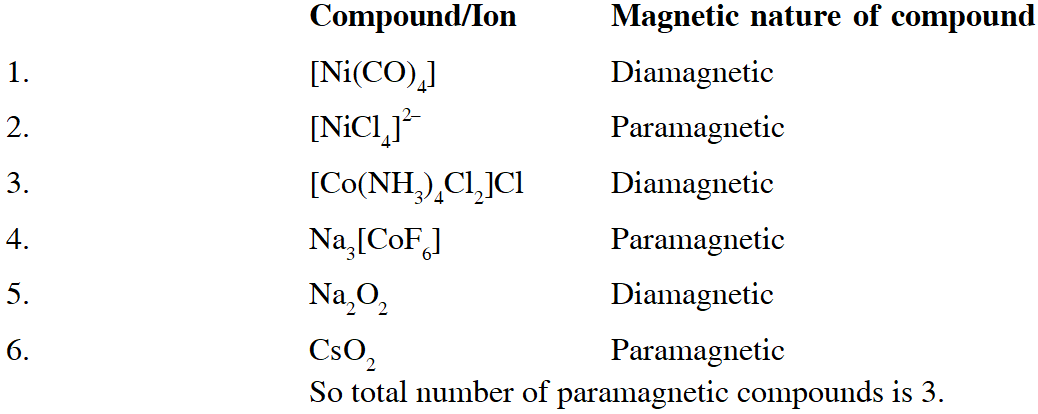Q. The number of geometric isomers possible for the complex$\left[\mathrm{CoL}_{2} \mathrm{Cl}_{2}\right]^{-}\left(\mathrm{L}=\mathrm{H}_{2} \mathrm{NCH}_{2} \mathrm{CH}_{2} \mathrm{O}^{-}\right)$ is [JEE - Adv. 2016]
Ans. 5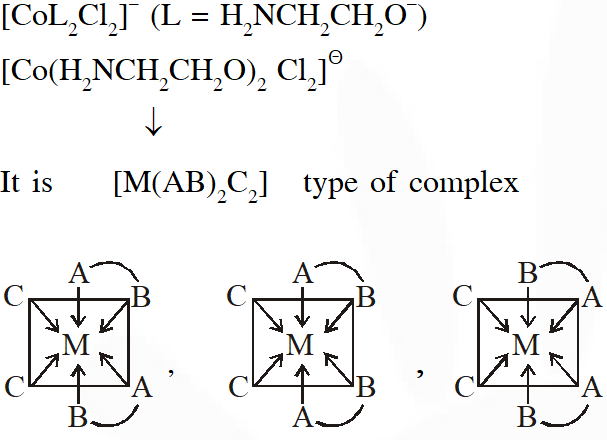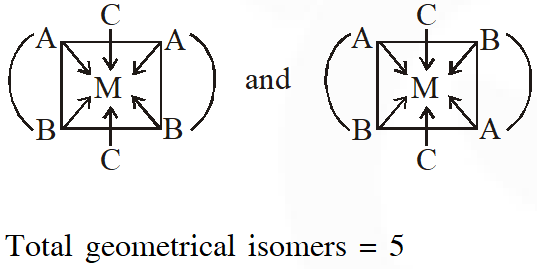Q. Addition of excess aqueous ammonia to a pink coloured aqueous solution of $\mathrm{MCl}_{2} \cdot 6 \mathrm{H}_{2} \mathrm{O}$ (X) and $\mathrm{NH}_{4} \mathrm{Cl}$ gives an octahedral complex Y in the presence of air. In aqueous solution, complex Y behaves as 1 : 3 electrolyte. The reaction of X with excess HCl at room temperature results in the formation of a blue coloured complex Z. The calculated spin only magnetic moment of X and Z is 3.87 B.M., whereas it is zero for complex Y. Among the following options, which statements is(are) correct ? (A) The hybridization of the central metal ion in Y is d2sp3 (B) Z is tetrahedral complex (C) Addition of silver nitrate to Y gives only two equivalents of silver chloride (D) When X and Z are in equilibrium at 0°C, the colour of the solution is pink [JEE - Adv. 2017]
Ans. (A,B,D)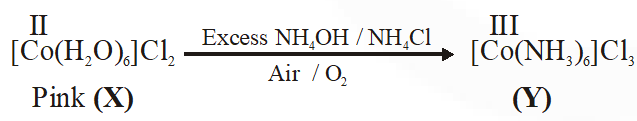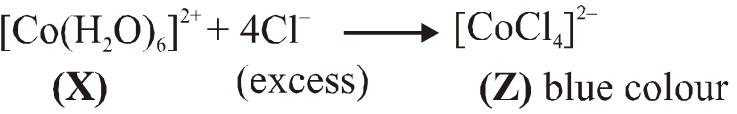(A) Hybridisation of $(\mathrm{Y})$ is $\mathrm{d}^{2} \mathrm{sp}^{3}$ as $\mathrm{NH}_{3}$ is strong field ligand (B) $\left[\mathrm{CoCl}_{4}\right]^{2-}$ have $\mathrm{sp}^{3}$ hybridisation as $\mathrm{Cl}^{-}$ is weak field ligand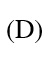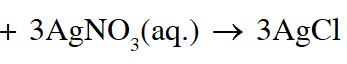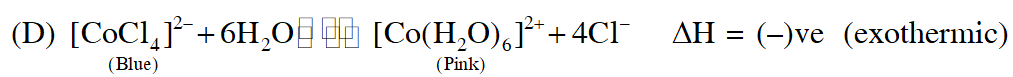When ice is added to the solution the equilibrium shifts right hence pink colour will remain predominant So, correct answer is (A,B& D)
A
Q. The correct statement(s) regarding the binary transition metal carbonyl compounds is (are) (Atomic numbers : Fe = 26, Ni = 28) (A) Total number of valence shell electrons at metal centre in $\mathrm{Fe}(\mathrm{CO})_{5}$ or $\mathrm{Ni}(\mathrm{CO})_{4}$ is 16 (B) These are predominantly low spin in nature (C) Metal - carbon bond strengthens when the oxidation state of the metal is lowered (D) The carbonyl C–O bond weakens when the oxidation state of the metal is increased [JEE - Adv. 2018]
Ans. (B,C) (A) $\left[\mathrm{Fe}\left(\mathrm{CO}_{5}\right)\right] \&\left[\mathrm{Ni}(\mathrm{CO})_{4}\right]$ complexes have 18-electrons in their valence shell. (B) Carbonyl complexes are predominantly low spin complexes due to strong ligand field. (C) As electron density increases on metals (with lowering oxidation state on metals), the extent of synergic bonding increases. Hence M–C bond strength increases (D) While positive charge on metals increases and the extent of synergic bond decreases and hence C–O bond becomes stronger.
Q. Among the species given below, the total number of diamagnetic species is____. H atom, $\mathrm{NO}_{2}$ monomer, $\mathrm{O}_{2}^{-}$ (superoxide), dimeric sulphur in vapour phase, $\mathrm{Mn}_{3} \mathrm{O}_{4},\left(\mathrm{NH}_{4}\right)_{2}\left[\mathrm{FeCl}_{4}\right],\left(\mathrm{NH}_{4}\right)_{2}\left[\mathrm{NiCl}_{4}\right], \mathrm{K}_{2} \mathrm{MnO}_{4}, \mathrm{K}_{2} \mathrm{CrO}_{4}$ [JEE - Adv. 2018]
Ans. (1)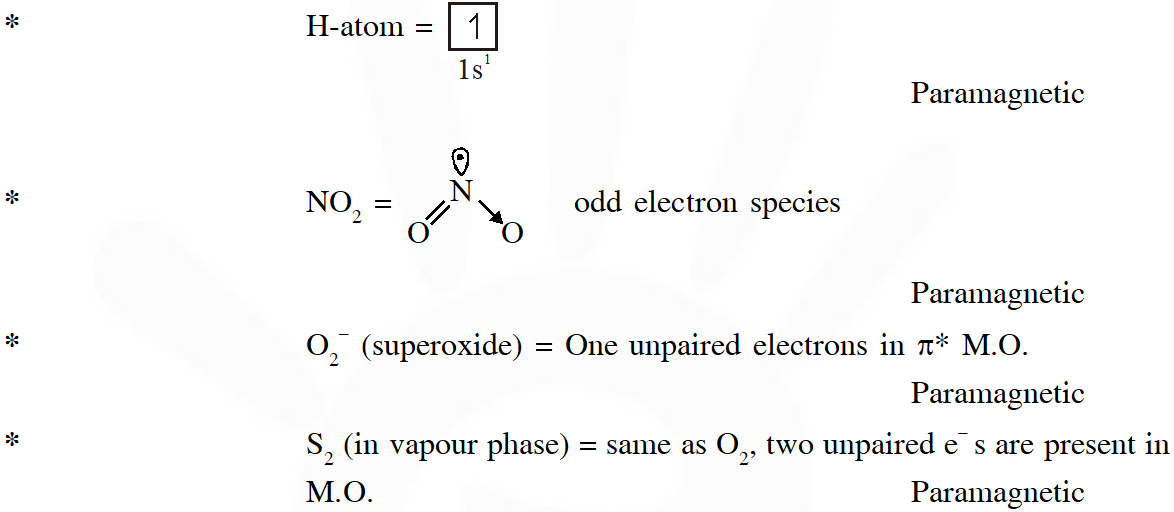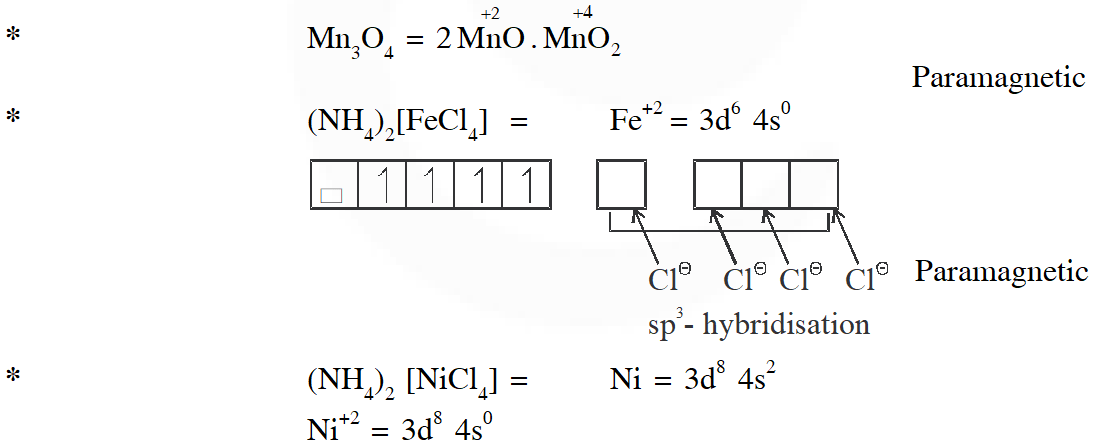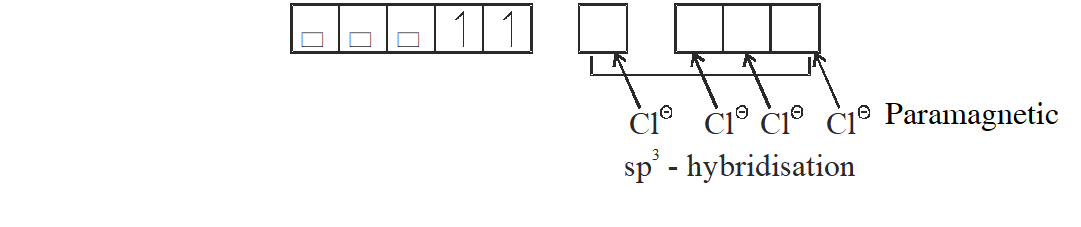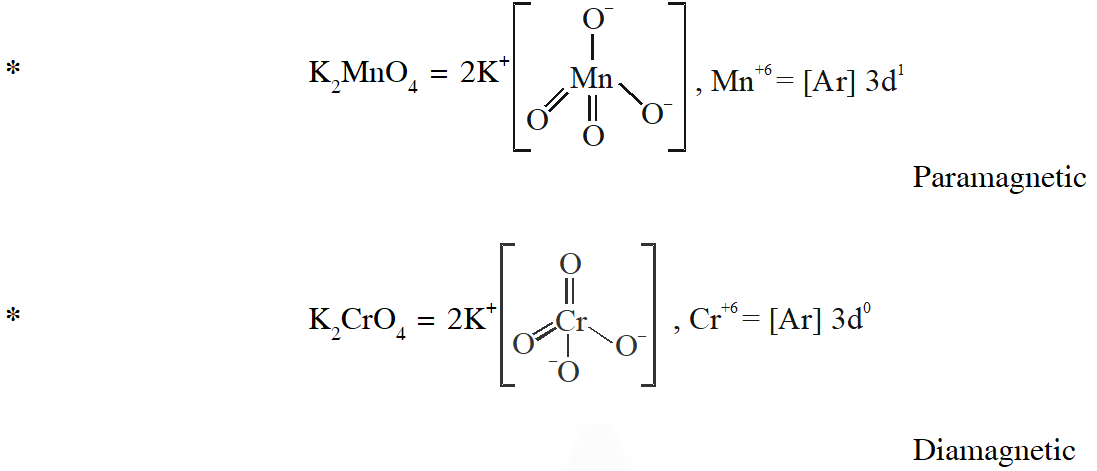Q. The ammonia prepared by treating ammonium sulphate with calcium hydroxide is completely used by $\mathrm{NiCl}_{2} \cdot 6 \mathrm{H}_{2} \mathrm{O}$ to form a stable coordination compound. Assume that both the reactions are 100% complete. If 1584 g of ammonium sulphate and 952g of NiCl2.6H2O are used in the preparation, the combined weight (in grams) of gypsum and the nickel-ammonia coordination compound thus produced is___. (Atomic weights in g $\mathrm{mol}^{-1}$: H = 1, N = 14, O = 16, S = 32, Cl = 35.5, Ca = 40, Ni = 59) (A) It has two geometrical isomers (B) It will have three geometrical isomers if bidentate 'en' is replaced by two cyanide ligands (C) It is paramagnetic (D) It absorbs light at longer wavelength as compared to $\left[\mathrm{Co}(\mathrm{en})\left(\mathrm{NH}_{3}\right)_{4}\right]^{3+}$ [JEE - Adv. 2018]
Ans. 2992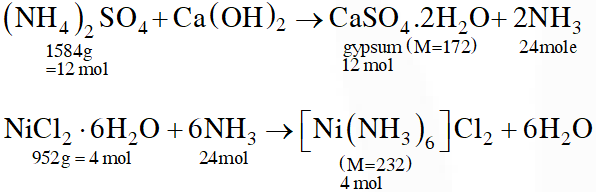Total mass = 12 × 172 + 4 × 232 = 2992 g
Q. The correct option(s) regarding the complex $\left[\mathrm{Co}(\mathrm{en})\left(\mathrm{NH}_{3}\right)_{3}\left(\mathrm{H}_{2} \mathrm{O}\right)\right]^{3+}:-$ $\left(\mathrm{en}=\mathrm{H}_{2} \mathrm{NCH}_{2} \mathrm{CH}_{2} \mathrm{NH}_{2}\right)$ is (are) [JEE - Adv. 2018]
Ans. (A,B,D)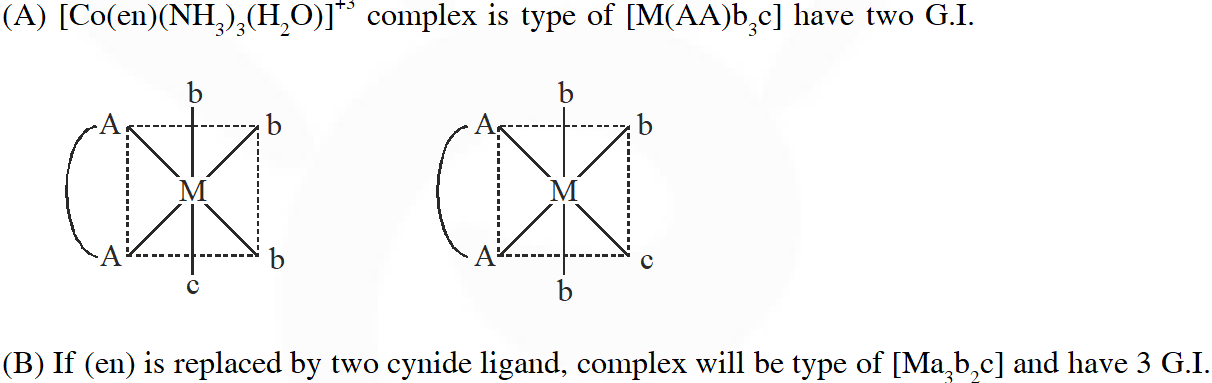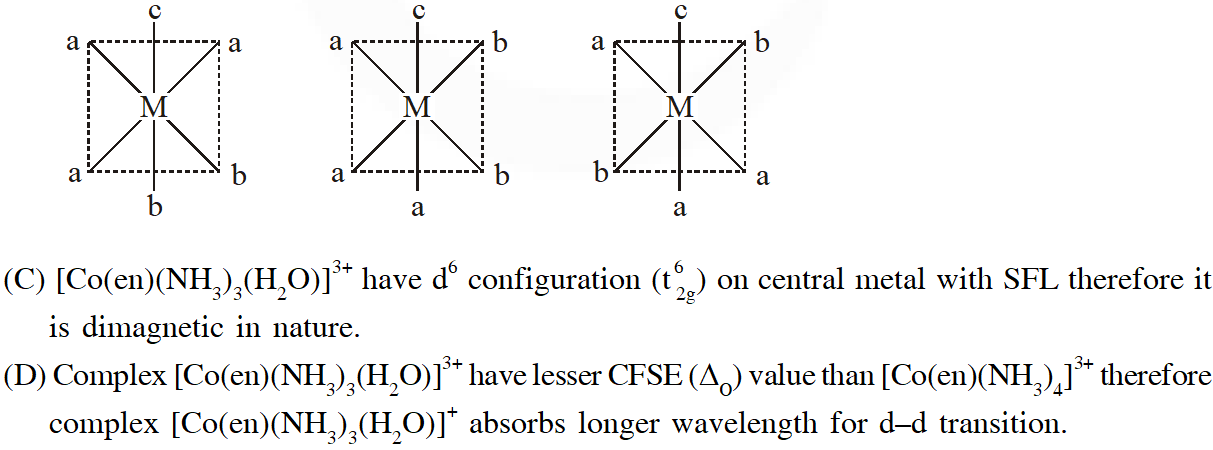Q. Match each set of hybrid orbitals from LIST-I with complex (es) given in LIST-II.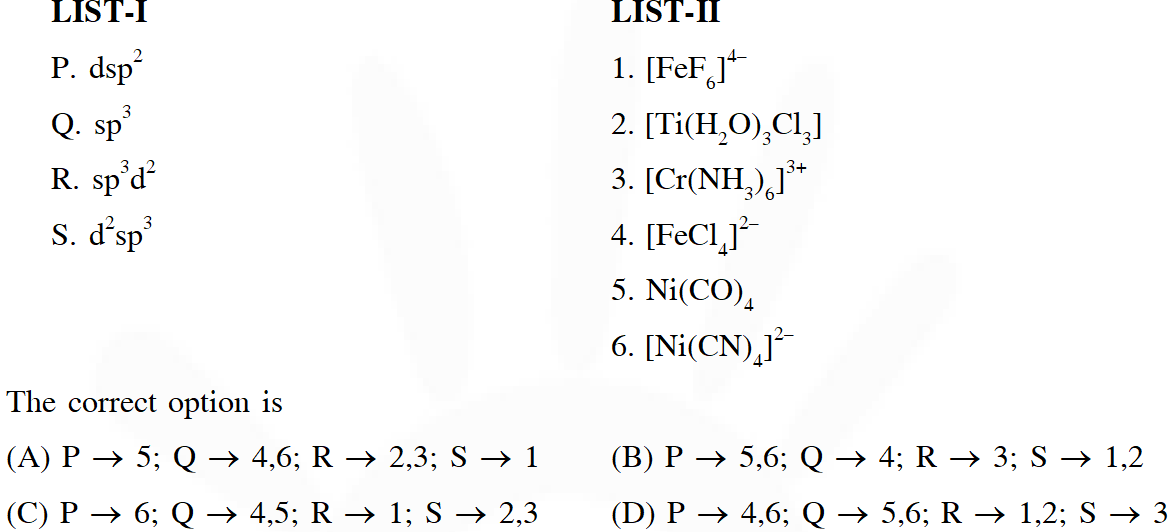[JEE - Adv. 2018]
Ans. (C)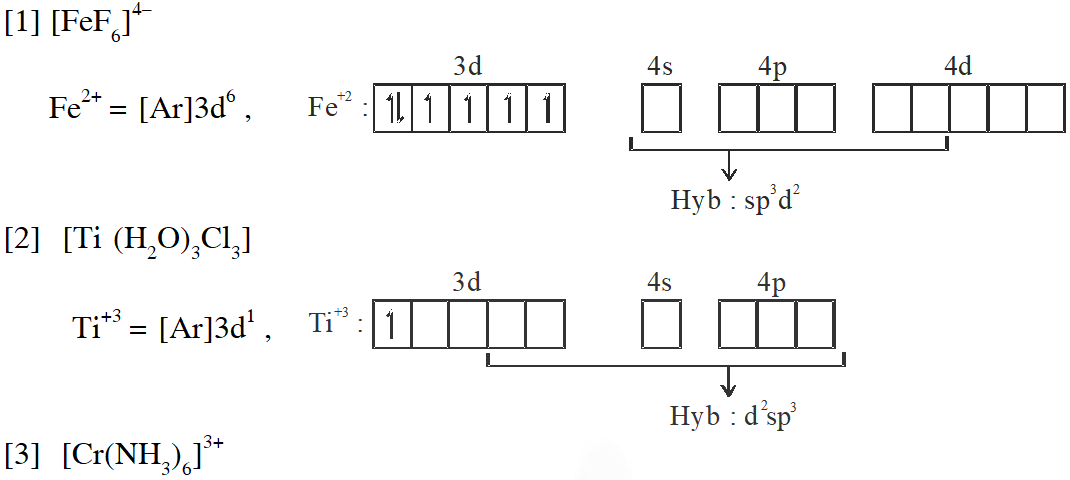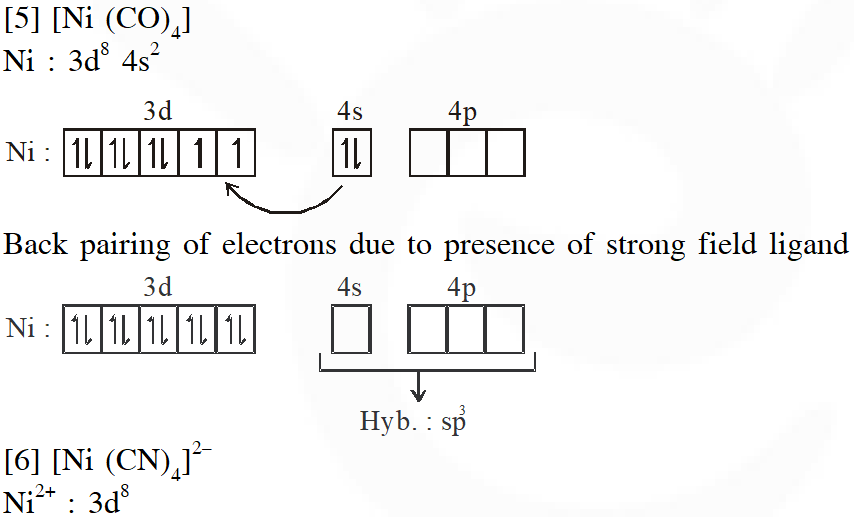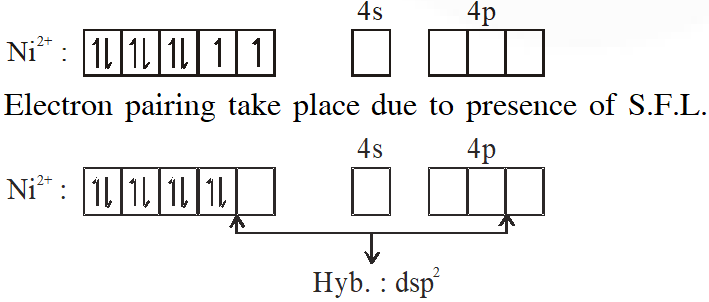pankaj
Aug. 20, 2022, 11:20 a.m.
Vipanchini Yanamala
Aug. 14, 2022, 6:18 p.m.
Providing solutions was helpful but few questions are incomplete
srujan ii bombay
Dec. 11, 2020, 2:21 p.m.
it was very helpful and made to know our mistakes before exam ..thank u
Sept. 26, 2020, 2:09 p.m.
oh no i not understand your paper plz do hard work
kalpana
Sept. 26, 2020, 10:56 a.m.
thanks
Bharath
Sept. 25, 2020, 11:02 a.m.
Thanks 😊😊😊😊😊
Viraj
Sept. 22, 2020, 11:26 p.m.
Nice revision..
kruthi
Sept. 19, 2020, 2:30 p.m.
super
PIYUSH
Sept. 16, 2020, 6:26 a.m.
I love this site and thanks for such a better solution and practice 😇😇
gaurav kumar
Sept. 15, 2020, 8:37 p.m.
Ruqqu
Sept. 13, 2020, 6:14 p.m.
Tysm
Manoj
Aug. 28, 2020, 12:56 p.m.
Thanks
Uma
Aug. 25, 2020, 4:46 p.m.
Nice
Ravi
June 18, 2020, 8:30 a.m.
The question which was given jee adv 2016 was not clear Thank you
neeraj
June 16, 2020, 3:52 p.m.
nice job
.
May 5, 2020, 11:51 p.m.
.
None
Free Study Material